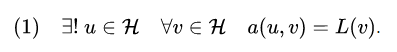# Power P integral variational formulation

Hello, I have a problem formulation in this (simplified) form :

Where u_primal is a known vector, and u_dual v_dual are test and trial functions.
In order to solve this problem on NGSolve, I use the Lax-Milgram theorem to change the weak formulation.
The aim is now to to solve this problem.

The way I did this is :

``````def run_dual(gfRho,gfu_primal):

gfu_dual = GridFunction(V2)

# Known value of the integral since u_primal is known
first_integral=([val**(1/exp - 1) for val in Integrate(gfRho * stress(gfu_primal) ,mesh, element_wise=True)])

# Lax Milgram formulation
aNRJ=BilinearForm(V2, symmetric=True,check_unused=False)

#  Right part of the formula (with epsilon(u) : epsilon(v))
#  Left part of the formula
aNRJ+=SymbolicEnergy(-1 *  first_integral   * stress(v_dual)  , definedon=mesh.Materials("active"))

# Solver
solveStateEquationEnergy(gfu_dual,4,aNRJ,V2, dampfactor=0.1,maxit=40,maxerr=0.5e-8)

return gfu_dual
``````

My question is the following : The way I implemented the first integrale (at the 1/exp - 1 power) is for sure wrong. I don’t have any clue on how to achieve it and write the exact same way my formula. Can you please help me to achieve implementing this formula ?
I am sure that the first part (left part of formula) is correct, the error is how I wrote the left part of the formula.

Thanks a lot !Your first term does not contain any test function? Or is there a typo and the u_dual should be a v_dual?

Why do you compute the integral element wise? From your formulation it seems you should use Integrate(rho * stress(gfuprimal), mesh)``````f = LinearForm(fes)